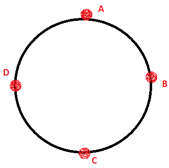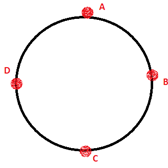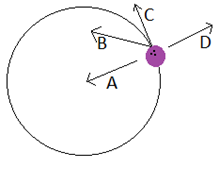Physics
Easy

Question

# What is the direction of the velocity at point mentioned if the ball is revolving anticlockwise?At point D

## UpDownLeftRightHint:

## The correct answer is: Down

Physics

Physics

Physics

### What is the direction of the velocity at point mentioned if the ball is revolving anticlockwise?At point APhysics

Physics

Physics

### An object is moving at a uniform speed in a counterclockwise, horizontal circle. Which of the following images correctly depicts the force and velocity at a point in time?Physics

Physics

Physics

### A boy moves a tied stone above his head in circles, time period of circular motion is 2 sec and it forms a circle of 20 cm radius. What is the speed of rotation?Physics

Physics

Physics

### What is the direction of the velocity at point mentioned if the ball is revolving clockwise.At point BPhysics

Physics

Physics

### Which of the following depicts the direction of velocity of the ball circulated around a point?#### With Turito Foundation.#### Get an Expert Advice From Turito.